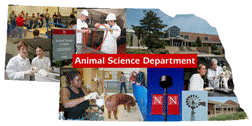Animal Science DepartmentFaculty Papers and Publications in Animal Science

Date of this Version

January 1987

Published in J Dairy Sci1987, 70:842-849. Copyright © 1987 The American Dairy Science Association. Used by permission.

Abstract

Genetic relationships among lactation records are of interest because most selection of bulls is on first lactations. Selection also complicates estimation of genetic parameters. Techniques unbiased by selection should be used. Estimation of genetic and environmental (co)variances was done using restricted maximum likelihood with an expectation-maximization algorithm for an animal model. The algorithm involved solving mixed model equations by direct inversion of coefficient matrix that became feasible by neglecting relationships across herds. From data consisting of first to third lactation milk records of New York Holsteins, two computationally manageable subsets were selected of 15 herds each totaling 3070 and 2900 cows. Each cow had a recorded first lactation and a recorded second lactation if she had a recorded third record. Herds were chosen according to frequency of related animals and about 200 cows per herd. After 18 rounds of iteration, changes in estimates between successive rounds were con- Stantly decreasing and small. Estimates averaged from both subsets gave heritabilities of h12 = .33, h22 = .33, h32 = .34, genetic correlations of rg12 = .86, rg13 = .85, rg23 = .87, and phenotypic correlations of rp12 = .57, rp13 = .52, rp23 = .65.

COinS